# Machine Learning Logistic Regression In Python: From Theory To Trading

In this blog post, we will learn how logistic regression works in machine learning for trading and will implement the same to predict stock price movement in Python.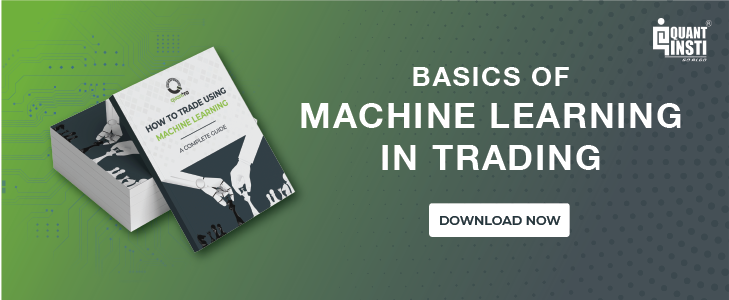Any machine learning tasks can roughly fall into two categories:

1. The expected outcome is defined
2. The expected outcome is not defined

The 1st one where the data consists of an input data and the labelled output is called supervised learning. The 2nd one where the datasets consisting of input data without labelled responses is called unsupervised learning. There is also another category called reinforcement learning that tries to retro-feed the model to improve performance.

### Logistic Regression and Linear regression

Logistic regression falls under the category of supervised learning; it measures the relationship between the categorical dependent variable and one or more independent variables by estimating probabilities using a logistic/sigmoid function. In spite of the name ‘logistic regression’, this is not used for machine learning regression problem where the task is to predict the real-valued output. It is a classification problem which is used to predict a binary outcome (1/0, -1/1, True/False) given a set of independent variables.

Logistic regression is a bit similar to the linear regression or we can say it as a generalized linear model. In linear regression, we predict a real-valued output 'y' based on a weighted sum of input variables.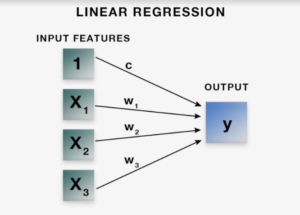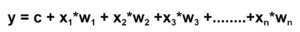The aim of linear regression is to estimate values for the model coefficients c, w1, w2, w3 ….wn and fit the training data with minimal squared error and predict the output y.

Logistic regression does the same thing, but with one addition. The logistic regression model computes a weighted sum of the input variables similar to the linear regression, but it runs the result through a special non-linear function, the logistic function or sigmoid function to produce the output y. Here, the output is binary or in the form of 0/1 or -1/1.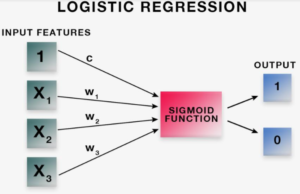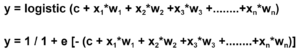The sigmoid/logistic function is given by the following equation.

y = 1 / 1+ e-x

As you can see in the graph, it is an S-shaped curve that gets closer to 1 as the value of input variable increases above 0 and gets closer to 0 as the input variable decreases below 0. The output of the sigmoid function is 0.5 when the input variable is 0.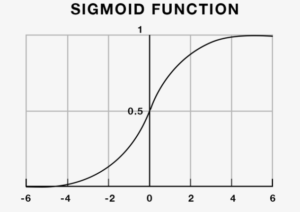Thus, if the output is more than 0.5, we can classify the outcome as 1 (or positive) and if it is less than 0.5, we can classify it as 0 (or negative).

Now, let us consider the task of predicting the stock price movement. If tomorrow’s closing price is higher than today’s closing price, then we will buy the stock (1), else we will sell it (-1). If the output is 0.7, then we can say that there is a 70% chance that tomorrow’s closing price is higher than today’s closing price and classify it as 1.

Now, we have a basic intuition behind the logistic regression and the sigmoid function. We will learn how to implement logistic regression in Python and predict the stock price movement using the above condition.

### Code Overview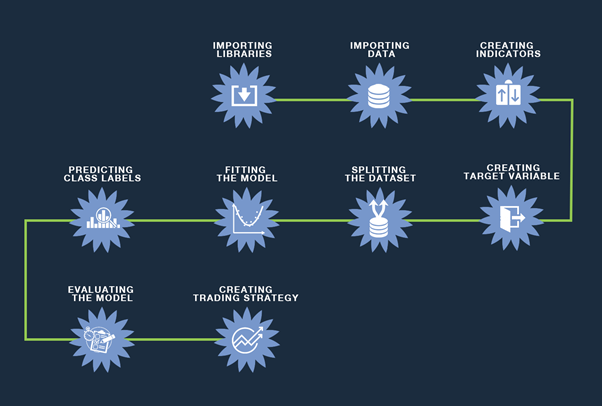#### Import The Libraries

We will start by importing the necessary libraries.

# Data Manipulation
import numpy as np
import pandas as pd

# Technical Indicators
import talib as ta

# Plotting graphs
import matplotlib.pyplot as plt

# Machine learning
from sklearn.linear_model import LogisticRegression
from sklearn import metrics
from sklearn.model_selection import cross_val_score

# Data fetching
from pandas_datareader import data as pdr
import yfinance as yf
yf.pdr_override()


#### Import The Data

We will import the Nifty 50 data from 01-Jan-2000 to 01-Jan-2018. The data is imported from yahoo finance using ‘pandas_datareader’.

df = pdr.get_data_yahoo('^NSEI', '2000-01-01', '2018-01-01')
df = df.dropna()
df = df.iloc[:,:4]


Let us print the top five rows of column ‘Open’, ‘High’, ‘Low’, ‘Close’.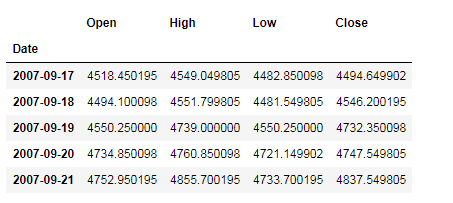#### Define Predictor/Independent Variables

We will use 10-days moving average, correlation, relative strength index (RSI), the difference between the open price of yesterday and today, difference close price of yesterday and the open price of today, open, high, low, and close price as indicators to make the prediction.

df['S_10'] = df['Close'].rolling(window=10).mean()
df['Corr'] = df['Close'].rolling(window=10).corr(df['S_10'])
df['RSI'] = ta.RSI(np.array(df['Close']), timeperiod =10)
df['Open-Close'] = df['Open'] - df['Close'].shift(1)
df['Open-Open'] = df['Open'] - df['Open'].shift(1)
df = df.dropna()
X = df.iloc[:,:9]


You can print and check all the predictor variables used to make a stock price prediction.

#### Define Target/Dependent Variable

The dependent variable is the same as discussed in the above example. If tomorrow’s closing price is higher than today’s closing price, then we will buy the stock (1), else we will sell it (-1).

y = np.where(df['Close'].shift(-1) > df['Close'],1,-1)

#### Split The Dataset

We will split the dataset into a training dataset and test dataset. We will use 70% of our data to train and the rest 20% to test. To do this, we will create a split variable which will divide the data frame in a 70-30 ratio. ‘Xtrain’ and ‘Ytrain’ are train dataset. ‘Xtest’ and ‘Ytest’ are the test dataset.

split = int(0.7*len(df))
X_train, X_test, y_train, y_test = X[:split], X[split:], y[:split], y[split:]


#### Instantiate The Logistic Regression in Python

We will instantiate the logistic regression in Python using ‘LogisticRegression’ function and fit the model on the training dataset using ‘fit’ function.

model = LogisticRegression()
model = model.fit (X_train,y_train)


#### Examine The Coefficients

pd.DataFrame(zip(X.columns, np.transpose(model.coef_)))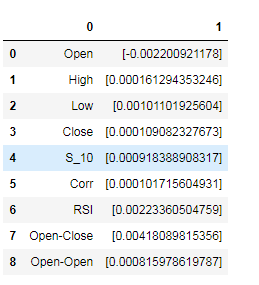#### Calculate Class Probabilities

We will calculate the probabilities of the class for the test dataset using ‘predict_proba’ function.

probability = model.predict_proba(X_test)
print probability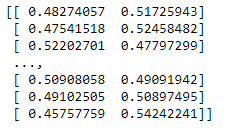#### Predict Class Labels

Next, we will predict the class labels using predict function for the test dataset.

probability = model.predict_proba(X_test)
print(probability)

predicted = model.predict(X_test)


If you print ‘predicted’ variable, you will observe that the classifier is predicting 1, when the probability in the second column of variable ‘probability’ is greater than 0.5. When the probability in the second column is less than 0.5, then the classifier is predicting -1.

### Evaluate The Model

#### Confusion Matrix

The Confusion matrix is used to describe the performance of the classification model on a set of test dataset for which the true values are known. We will calculate the confusion matrix using ‘confusion_matrix’ function.

print(metrics.confusion_matrix(y_test, predicted))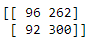You can interpret the above matrix as: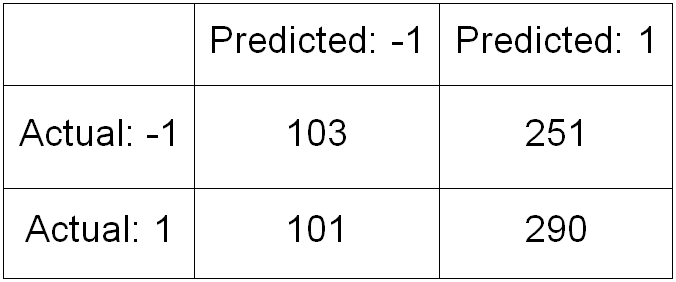#### Classification Report

This is another method to examine the performance of the classification model.

print(metrics.classification_report(y_test, predicted))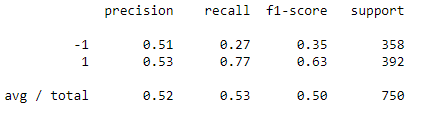The f1-score tells you the accuracy of the classifier in classifying the data points in that particular class compared to all other class. It is calculated by taking the harmonic mean of precision and recall. The support is the number of samples of the true response that lies in that class.

#### Model Accuracy

We will calculate the model accuracy on the test dataset using ‘score’ function.

print(model.score(X_test,y_test))
0.528

We can see the accuracy of 52%.

#### Cross-Validation

We will cross-check the accuracy of the model using 10-fold cross-validation. For this, we will use ‘crossvalscore’ function which we have imported from ‘sklearn.cross_validation’ library.

cross_val = cross_val_score(LogisticRegression(), X, y, scoring='accuracy', cv=10)
print(cross_val)
print(cross_val.mean())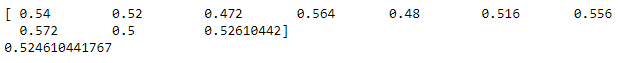The accuracy is still 52% which means the model is working fine.

#### Create Trading Strategy Using The Model

We will predict the signal to buy (1) or sell (-1) and calculate the cumulative Nifty 50 returns for the test dataset. Next, we will calculate the cumulative strategy return based on the signal predicted by the model in the test dataset. We will also plot the cumulative returns.

df['Predicted_Signal'] = model.predict(X)
df['Nifty_returns'] = np.log(df['Close']/df['Close'].shift(1))
Cumulative_Nifty_returns = np.cumsum(df[split:]['Nifty_returns'])

df['Startegy_returns'] = df['Nifty_returns']* df['Predicted_Signal'].shift(1)
Cumulative_Strategy_returns = np.cumsum(df[split:]['Startegy_returns'])

plt.figure(figsize=(10,5))
plt.plot(Cumulative_Nifty_returns, color='r',label = 'Nifty Returns')
plt.plot(Cumulative_Strategy_returns, color='g', label = 'Strategy Returns')
plt.legend()
plt.show()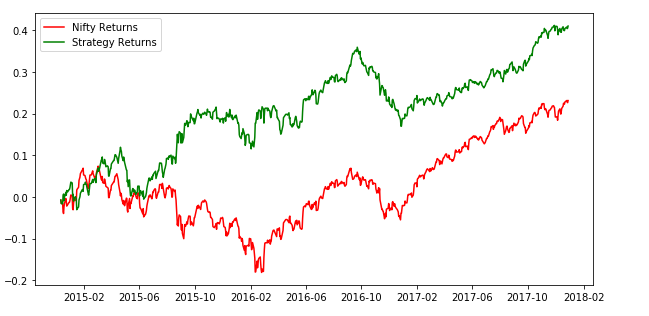### Conclusion

It can be observed that the Logistic Regression model in Python predicts the classes with an accuracy of approximately 52% and generates good returns. Now it’s your turn to play with the code by changing parameters and create a trading strategy based on it.

Want to know how to trade using machine learning in python? This blog will explain machine learning that can help new tool to generate more alpha with one such module.

Update - We have noticed that some users are facing challenges while downloading the market data from Yahoo and Google Finance platforms. In case you are looking for an alternative source for market data, you can use Quandl for the same.

Disclaimer: All investments and trading in the stock market involve risk. Any decisions to place trades in the financial markets, including trading in stock or options or other financial instruments is a personal decision that should only be made after thorough research, including a personal risk and financial assessment and the engagement of professional assistance to the extent you believe necessary. The trading strategies or related information mentioned in this article is for informational purposes only.